Ex 7.3

Chapter 7 Class 8 Comparing Quantities
Serial order wise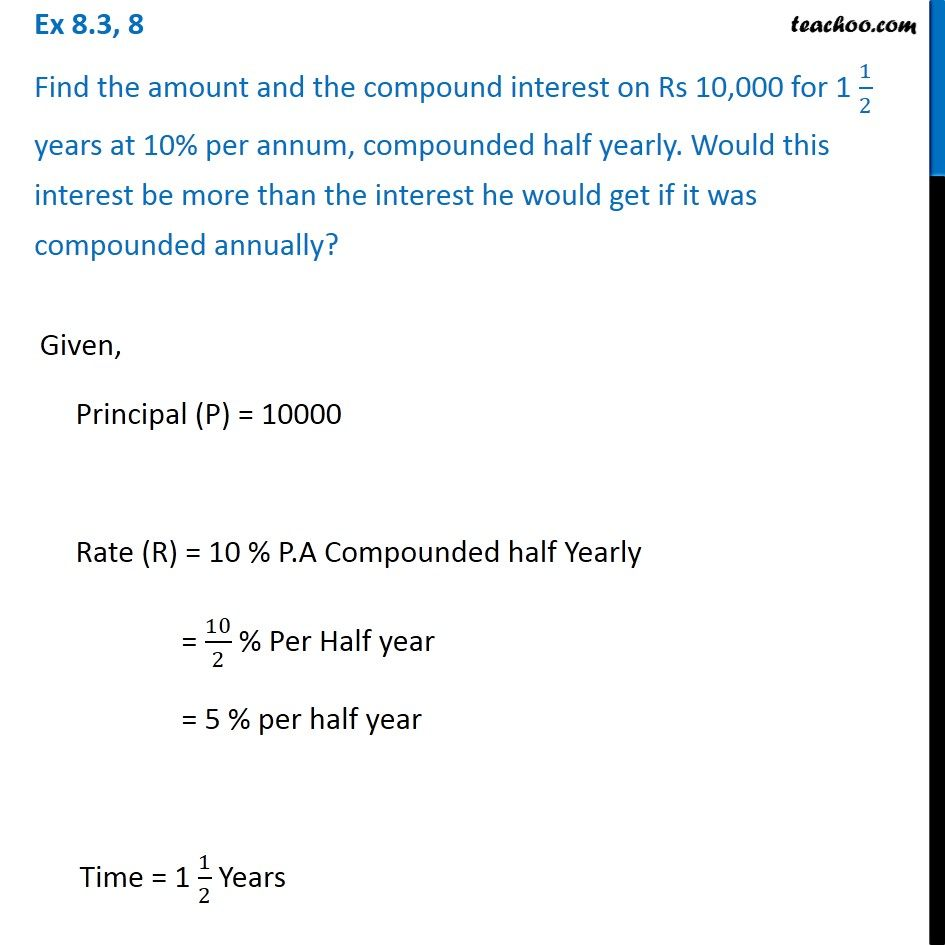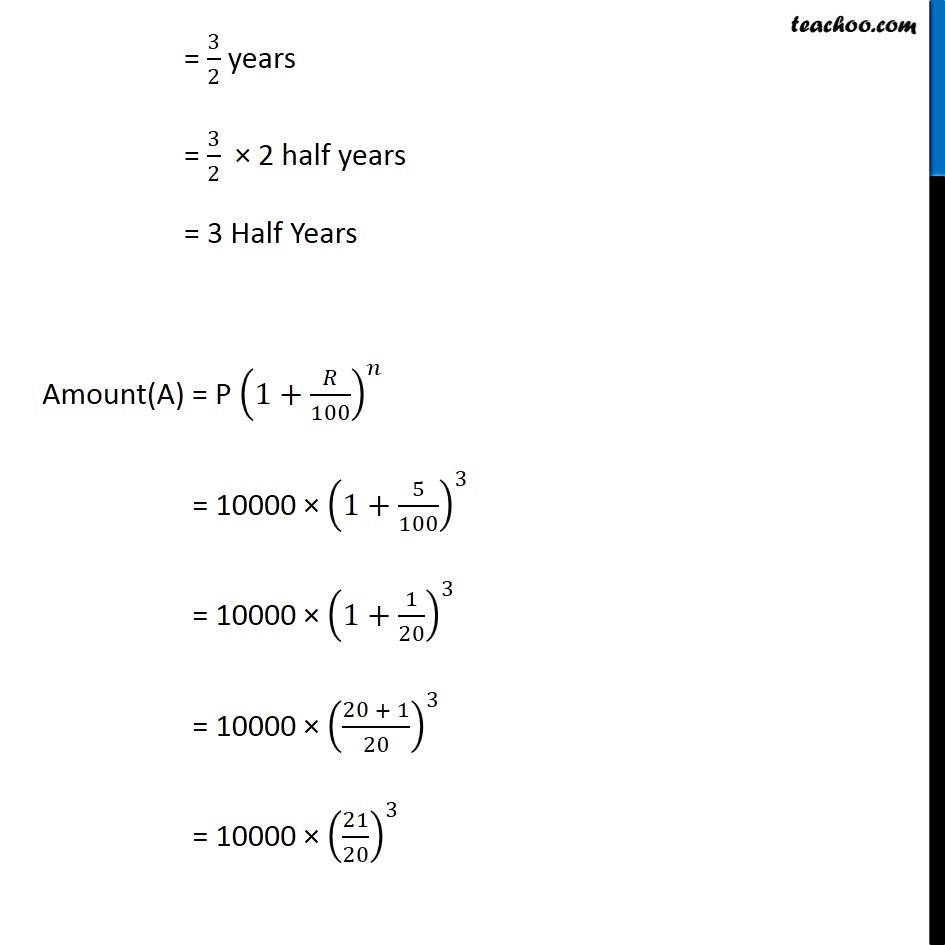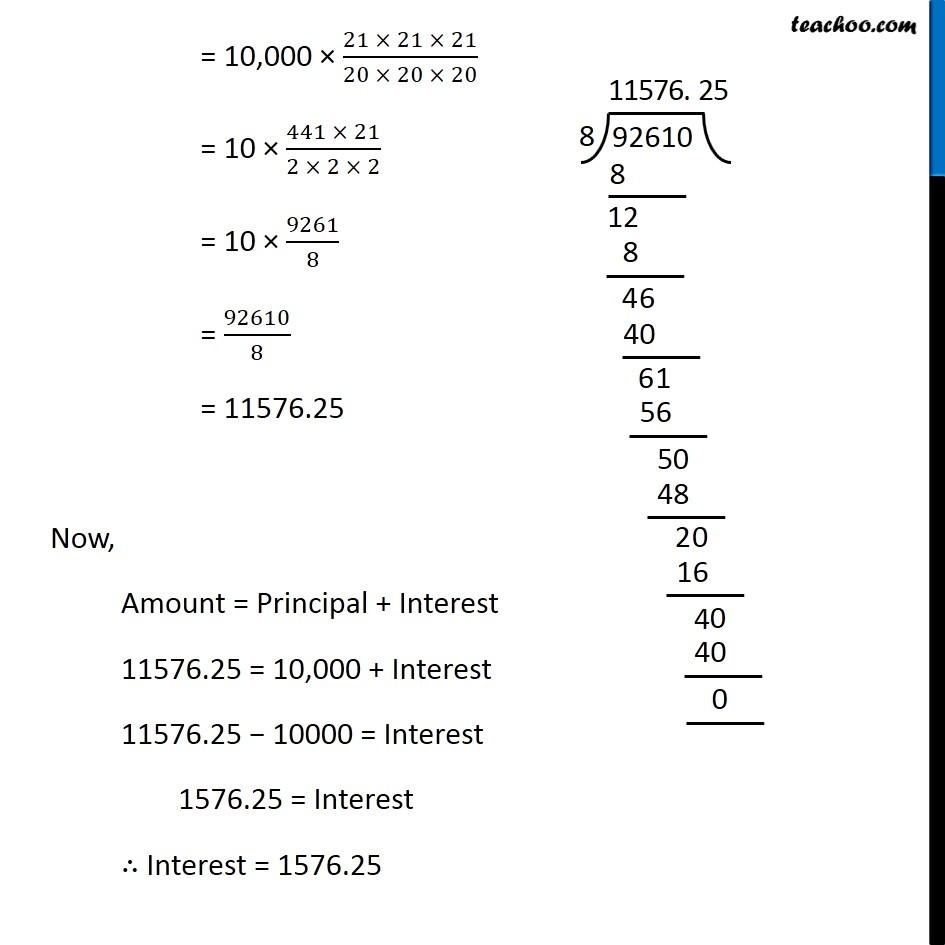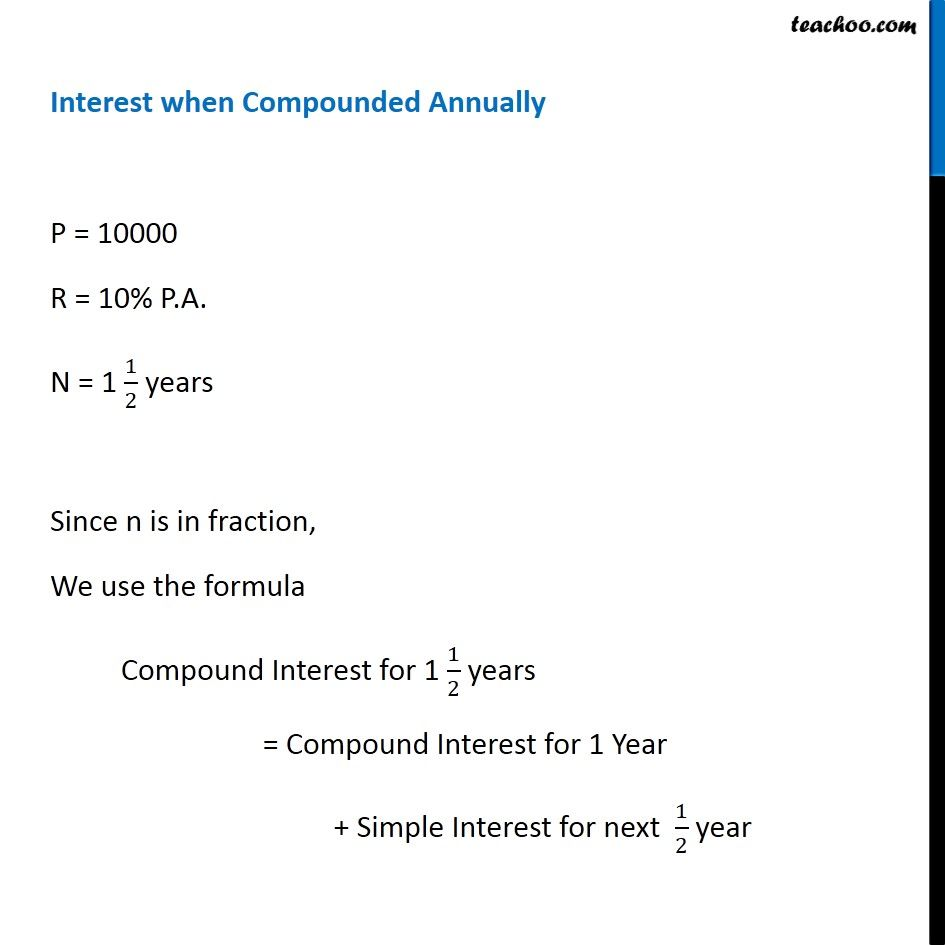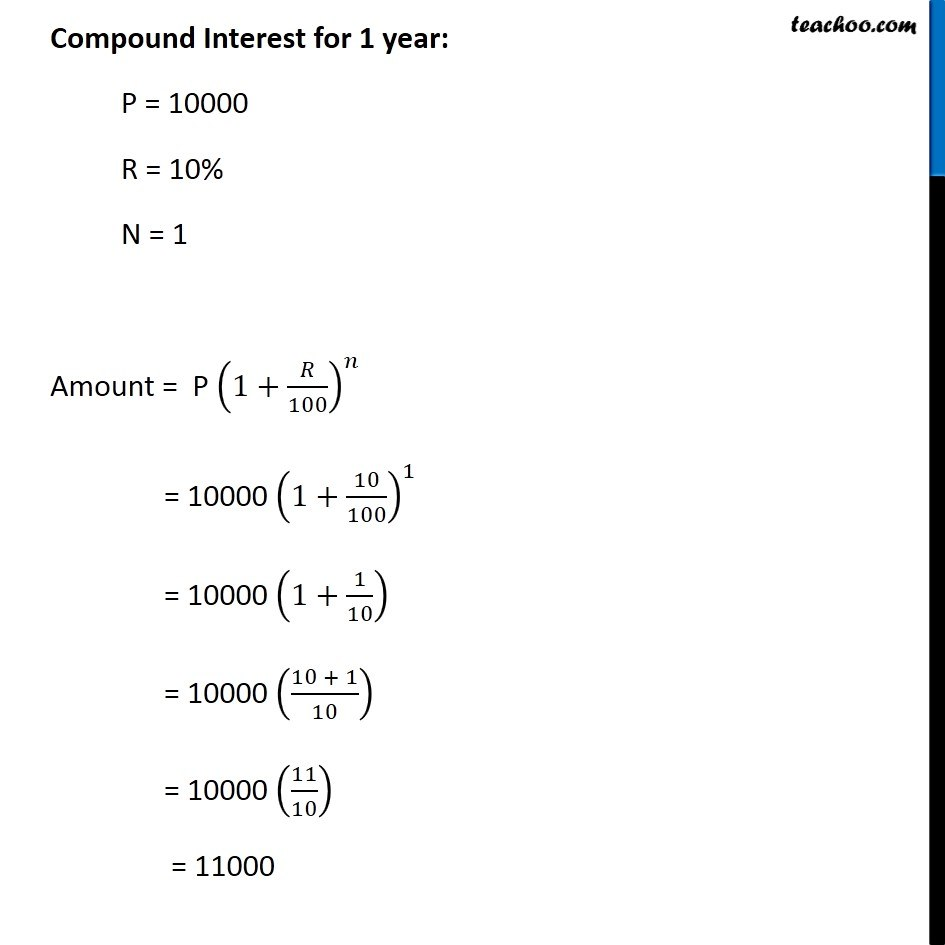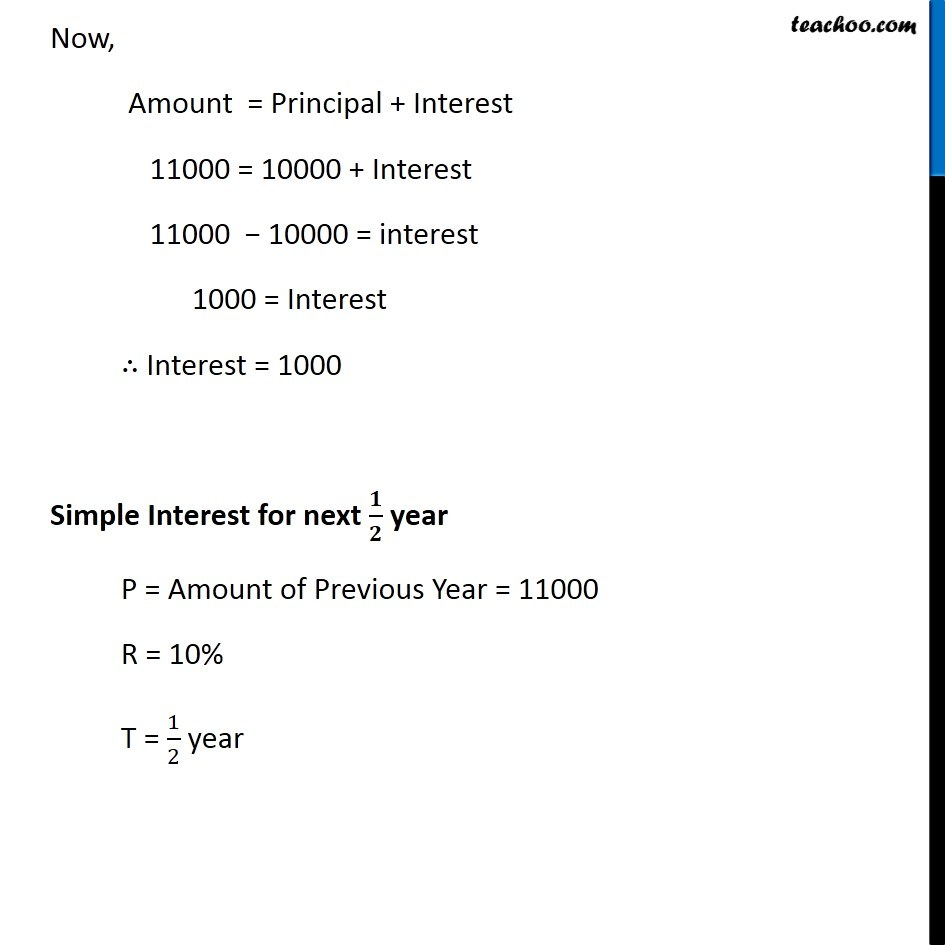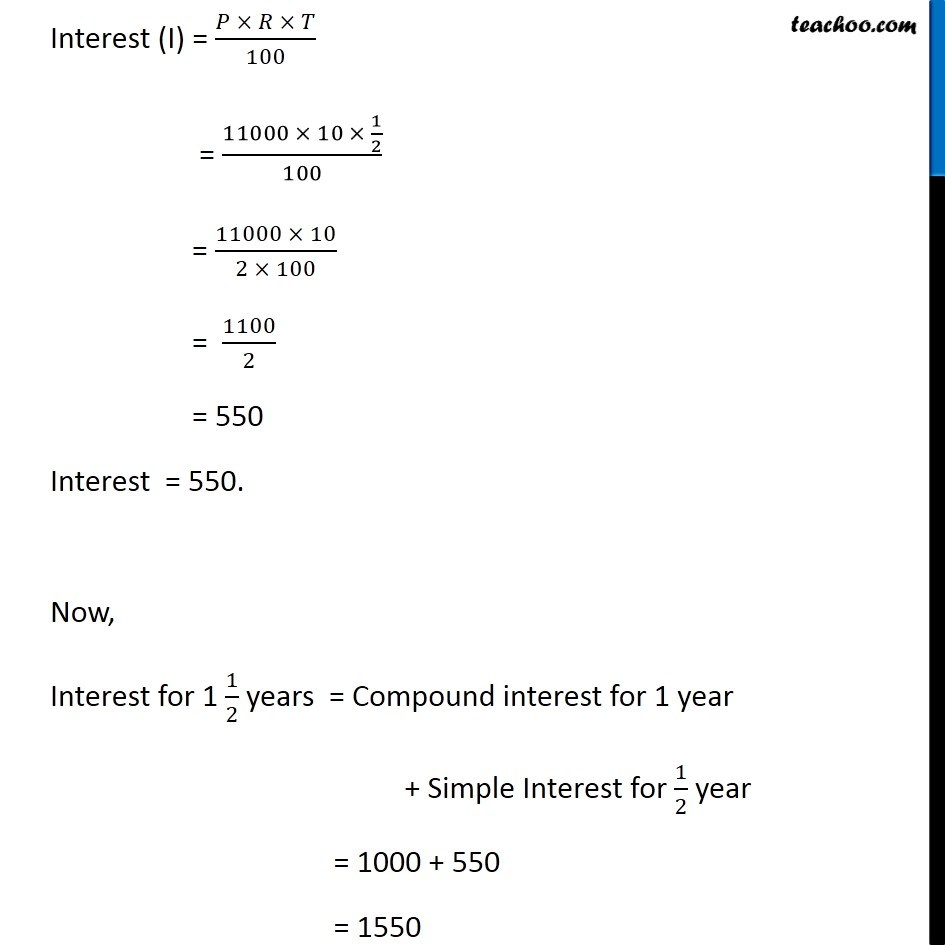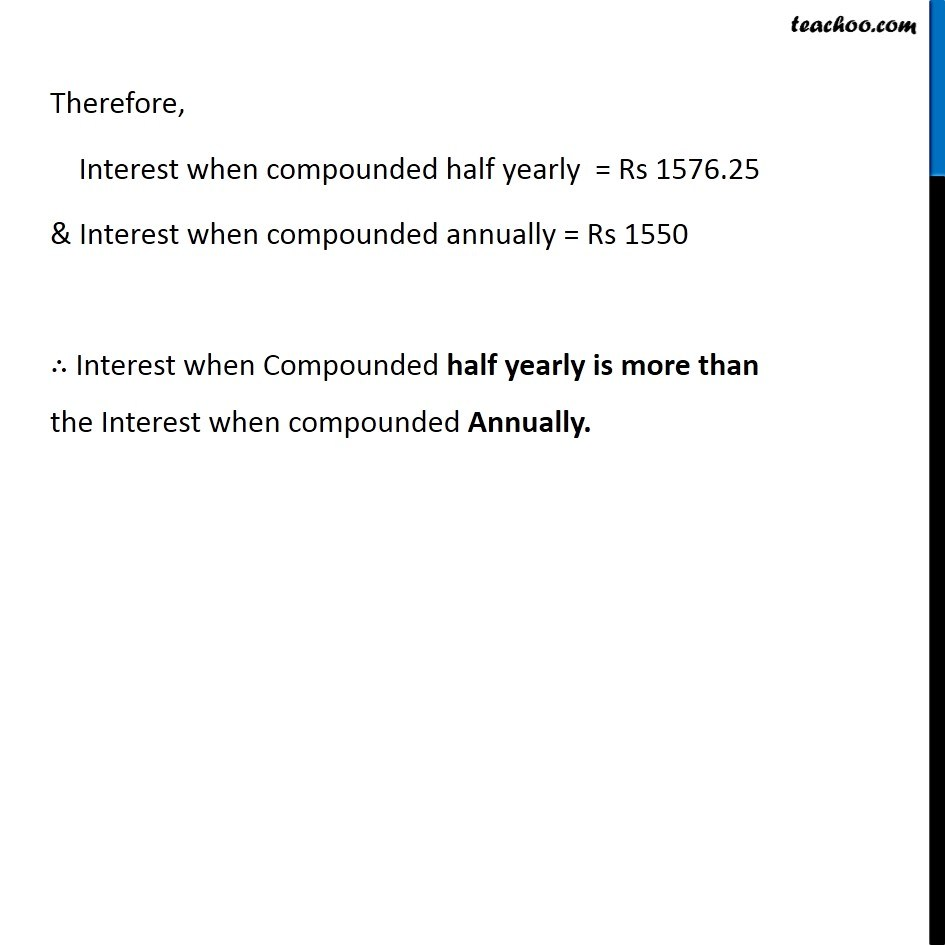Learn in your speed, with individual attention - Teachoo Maths 1-on-1 Class

### Transcript

Question 8 Find the amount and the compound interest on Rs 10,000 for 1 1/2 years at 10% per annum, compounded half yearly. Would this interest be more than the interest he would get if it was compounded annually? Given, Principal (P) = 10000 Rate (R) = 10 % P.A Compounded half Yearly = 10/2 % Per Half year = 5 % per half year Time = 1 1/2 Years = 3/2 years = 3/2 × 2 half years = 3 Half Years Amount(A) = P (1+𝑅/100)^𝑛 = 10000 × (1+5/100)^3 = 10000 × (1+1/20)^3 = 10000 × ((20 + 1)/20)^3 = 10000 × (21/20)^3 = 10,000 × (21 × 21 × 21)/(20 × 20 × 20) = 10 × (441 × 21)/(2 × 2 × 2) = 10 × 9261/8 = 92610/8 = 11576.25 Now, Amount = Principal + Interest 11576.25 = 10,000 + Interest 11576.25 − 10000 = Interest 1576.25 = Interest ∴ Interest = 1576.25 Interest when Compounded Annually P = 10000 R = 10% P.A. N = 1 1/2 years Since n is in fraction, We use the formula Compound Interest for 1 1/2 years = Compound Interest for 1 Year + Simple Interest for next 1/2 year Compound Interest for 1 year: P = 10000 R = 10% N = 1 Amount = P (1+𝑅/100)^𝑛 = 10000 (1+10/100)^1 = 10000 (1+1/10) = 10000 ((10 + 1)/10) = 10000 (11/10) = 11000 Now, Amount = Principal + Interest 11000 = 10000 + Interest 11000 − 10000 = interest 1000 = Interest ∴ Interest = 1000 Simple Interest for next 𝟏/𝟐 year P = Amount of Previous Year = 11000 R = 10% T = 1/2 year Interest (I) = (𝑃 × 𝑅 × 𝑇)/100 = (11000 × 10 × 1/2)/100 = (11000 × 10)/(2 × 100) = 1100/2 = 550 Interest = 550. Now, Interest for 1 1/2 years = Compound interest for 1 year + Simple Interest for 1/2 year = 1000 + 550 = 1550 Therefore, Interest when compounded half yearly = Rs 1576.25 & Interest when compounded annually = Rs 1550 ∴ Interest when Compounded half yearly is more than the Interest when compounded Annually.